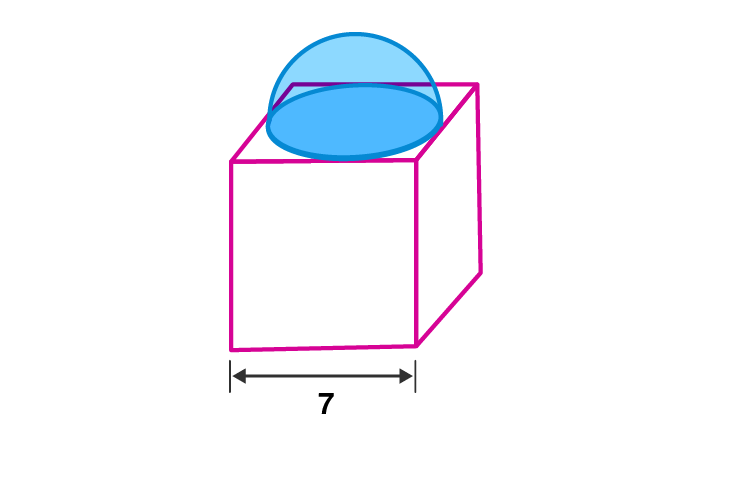Newbie

# A cubical block of side 7 cm is surmounted by a hemisphere. What is the greatest diameter that the hemisphere can have? Also, find the surface area of the solid.

• 0

ICSE Board Question Based on Mensuration Chapter of M.L Aggarwal for class10
A cubical block side is surmounted by a hemisphere. What is the greatest diameter that the hemisphere can have? Also, find the surface area of the solid.
This is the Question Number 07, Exercise 17.4 of M.L Aggarwal.

Share

1.Given edge of the cube, a = 7 cm

Diameter of the hemisphere, d = 7 cm

Radius, r = d/2 = 7/2 = 3.5 cm

Surface area of the hemisphere = 2r2

= 2×(22/7)×3.52

= 44×12.25/7

= 539/7

= 77 cm2

Surface area of the cube = 6a2

= 6×72

= 6×49

= 294 cm2

Surface area of base of hemisphere = r2

= (22/7)×3.52

= 22×12.25/7

= 38.5 cm2

Surface area of the solid = surface area of the cube + surface area of hemisphere – surface area of the base of hemisphere

= 294+77-38.5

= 332.5 cm2

Hence the surface area of the solid is 332.5 cm2.

• 0